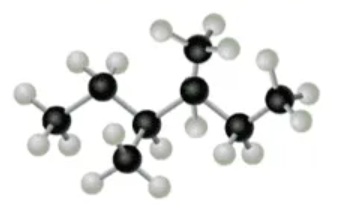# Problem: A.  What is the molecular formula for the alkane shown in the model? Express your answer as a chemical formula.B. Name the alkane shown in the model. Express your answer as the IUPAC name.

###### FREE Expert Solution

We’re being asked to determine the molecular formula and the name for the alkane shown in the model.

Alkanes are compounds that contain only carbons and hydrogens single bonded to one another. They are sometimes referred to simply as “hydrocarbons".Based on the model:

black spheres = carbon

white spheres = hydrogen

94% (345 ratings)###### Problem Details

A.  What is the molecular formula for the alkane shown in the model? Express your answer as a chemical formula.

B. Name the alkane shown in the model. Express your answer as the IUPAC name.